# Operations on Symbolic Expressions Guide

Task: get basic knowledge of how to work with Symbolic Toolbox in the MATLAB software package (Part 2).

Operations on Symbolic Expressions

Once an expression or variable is declared as a symbolic object, we can perform standard mathematical operations such as given in the table below.

 Function Description Function Description horner(S) transforms S into the Horner nested polynomial format [N,D] = numden(S) returns two symbolic numerator and denominator expressions sym(S,’?’); where ? may be f,r,e, or d symbolic to numeric conversion: f is floating point, r = rational form, e = rational plus an error term, d = decimal expansion when followed by digits(N) poly2sym(c) converts the polynomial vector c into a symbolic polynomial pretty(S) produces a typeset type display A+B symbolic addition of A+B A/B symbolic division of A/B A*B symbolic multiplication of AB S^p symbolic power S^p A-B symbolic subtraction A-B

Example: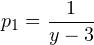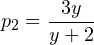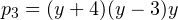syms y
p1 = 1/(y-3);
p2 = 3*y/(y+2);
p3 = (y+4) *(y-3) *y;

% Now operate: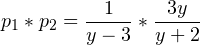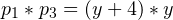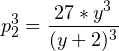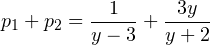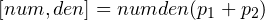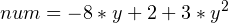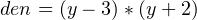Solutions to Equations

To solve a single equation, we use: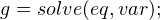That is, find what value of the independent variable makes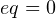; var is optional.

– If more than one variable is present, the variable to solve for set with var is: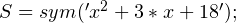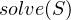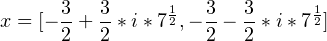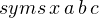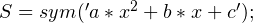% Contains four variables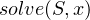% Solve for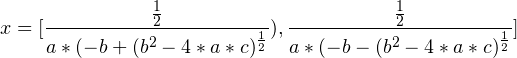To solve a system of equations, use: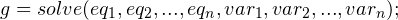The var_{1}, …,var_{n}are the variables to solve for. They must be declared as symbolics using syms.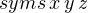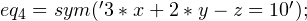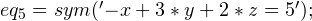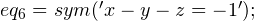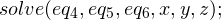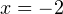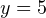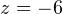When you get an assignment on a similar topic, such a sample can help you to deal with it. However, only a few students can complete really great tasks. To solve your problem, it is necessary to have someone who can help you. We will assist you with any problem; you just need to visit assignmentshark.com and leave your order. Therefore, you will get qualified help from the expert in the field. For better understanding of how our experts deal with different tasks, you can check out samples on our blog. We have samples presented for different disciplines.

On our site, you can find help with any type of task you may get. Our ordering system is easy and understandable for everyone. If you decide to order on our site, you can be sure that your assignment will contain all formulas and calculations that you need. The more assistance you get from us, the better your grades will be!